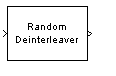Documentation

# Random Deinterleaver

Restore ordering of input symbols using random permutation

## Library

Block sublibrary of Interleaving## Description

The Random Deinterleaver block rearranges the elements of its input vector using a random permutation. The Initial seed parameter initializes the random number generator that the block uses to determine the permutation. If this block and the Random Interleaver block have the same value for Initial seed, then the two blocks are inverses of each other.

This block accepts a column vector input signal. The Number of elements parameter indicates how many numbers are in the input vector.

The block accepts the following data types `int8`, `uint8`, `int16`, `uint16`, `int32`, `uint32`, `boolean`, `single`, `double`, and fixed-point. The output signal inherits its data type from the input signal.

## Parameters

Number of elements

The number of elements in the input vector.

Initial seed

The initial seed value for the random number generator.

## Pair Block

Random Interleaver